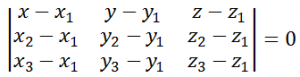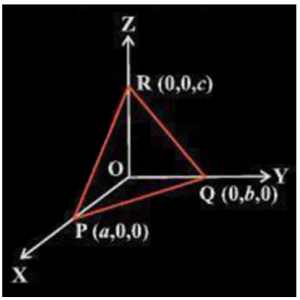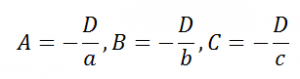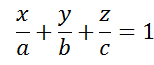# Equation of Plane - Intercept Form

Vectors are physical quantities which like other quantities have a magnitude but also a direction linked to them. In the three-dimensional Cartesian system, position vectors are simply used to denote the location or position of the point, but a reference point is necessary.

## Intercept form of the Equation of the Plane

There are infinite number of planes which are perpendicular to a particular vector as we have already discussed in our earlier sections. But when talking of a specific point only one exclusive plane occurs which is perpendicular to the point going through the given area. This can be denoted by this particular vector equation:

(

$$\begin{array}{l}\vec{r} – \vec{a} \end{array}$$
).
$$\begin{array}{l}\vec{N} \end{array}$$

Here,

$$\begin{array}{l}\vec{r} \end{array}$$
and
$$\begin{array}{l}\vec{a} \end{array}$$
denote the position vector

The denotation of this type of plane in a Cartesian equation is the following:

$$\begin{array}{l}A(x-{x}_{1}) + B (y- {y}_{1}) + C (z -{z}_{1}) = 0 \end{array}$$

The direction ratios here are denoted by A, B, and C.

Also the equation of a plane crossing the three non-collinear points in vector form is given as:

$$\begin{array}{l}(\vec{r} – \vec{a}).[(\vec{b}- \vec{a}) × (\vec{c}- \vec{a})] = 0 \end{array}$$

The equation of a plane in Cartesian form passing through three non-collinear points is given as:Let us now discuss the equation of a plane in intercept form.

The general equation of a plane is given as:

Ax + By + Cz + D = 0 (D ≠ 0)

Let us now try to determine the equation of a plane in terms of the intercepts which is formed by the given plane on the respective co-ordinate axes. Let us assume that the plane makes intercepts of a, b and c on the three co-ordinate axes respectively. Thus, the coordinates of the point of intersection of the plane with x, y and z axes are given by (a, 0, 0), (0, b, 0) and (0, 0, c) respectively.Substituting these values in the general equation of a plane, we have

Aa + D = 0

Bb + D = 0

Cc + D = 0

From the above three equations, we haveSubstituting these values of A, B, c and D in the general equation of the plane, we haveThis gives us the required equation of a plane in the intercept form.